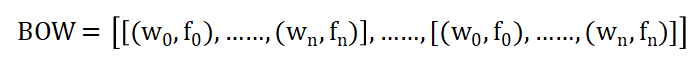4294字，阅读需时15分钟1、掌握词袋模型；

2、编写生成词袋模型的程序。

词袋模型dictionaries = {'计算机': 0, 'Java编程': 1, 'C++编程': 2, '编程': 3, 'Python编程': 4, '语言': 5, '课程': 6}
text = [[‘Java编程’,’课程’,’Python编程’,’课程’],[‘C++编程’,’语言’,’课程’]]

text依据字典dictionaries生成的词袋模型为：

BOW = [[(0,0),(1,1),(2,0),(3,0),(4,1),(5,0),(6,2)],
[(0,0),(1,0),(2,1),(3,0),(4,0),(5,1),(6,1)]]

dictionaries =  {'计算机': 0,
'Java编程': 1,
'C++编程': 2,
'编程': 3,
'Python编程': 4,
'语言': 5,
'课程': 6}

texts = [["Java编程","课程","Python编程","课程"],
['C++编程','语言','课程']]

# 应用字典corpus_dict转换text为词袋模型
def toBow(texts, corpus_dict):
# 存储词袋数据
bow = []
# 遍历文档序列
for text in texts:
v = []
# 遍历字典全部单词
for key in corpus_dict.keys():
if key in text:
'''
若text包含字典单词,创建二元组(数字ID,词频)
添加二元组到词袋
'''
v.append((corpus_dict[key], text.count(key)))
else:
'''
若text不包含字典单词,创建二元组(数字ID,0)
添加二元组到词袋
'''
v.append((corpus_dict[key], 0))
# 词袋数据按二元组的数字ID排序
v = sorted(v, key= lambda x: x)
bow.append(v)
return bow

# 程序入口
if __name__ == '__main__':
print(toBow(texts,dictionaries))

BOW = [[(0,0),(1,1),(2,0),(3,0),(4,1),(5,0),(6,2)],
[(0,0),(1,0),(2,1),(3,0),(4,0),(5,1),(6,1)]]

VBOW = [[0,1,0,0,1,0,2],
[0,0,1,0,0,1,1]]

# 词袋模型抽取为向量

def bow2Vector(bow):
vect = []
for m in bow:
v = []
for n in m:
v.append(n)
vect.append(v)
return vect

# 输出词袋模型
def doc2bow(self,texts):
# 存储词袋数据
self.bow = []
# 遍历文档序列
for text in texts:
v = []
# 遍历字典全部单词
for key in self.token2id.keys():
if key in text:
'''
若text包含字典单词,创建二元组(数字ID,词频)
添加二元组到词袋
'''
v.append((self.token2id[key], text.count(key)))
else:
'''
若text不包含字典单词,创建二元组(数字ID,0)
添加二元组到词袋
'''
v.append((self.token2id[key], 0))
# 词袋数据按二元组的数字ID排序
v = sorted(v, key= lambda x: x)
self.bow.append(v)
return self.bow